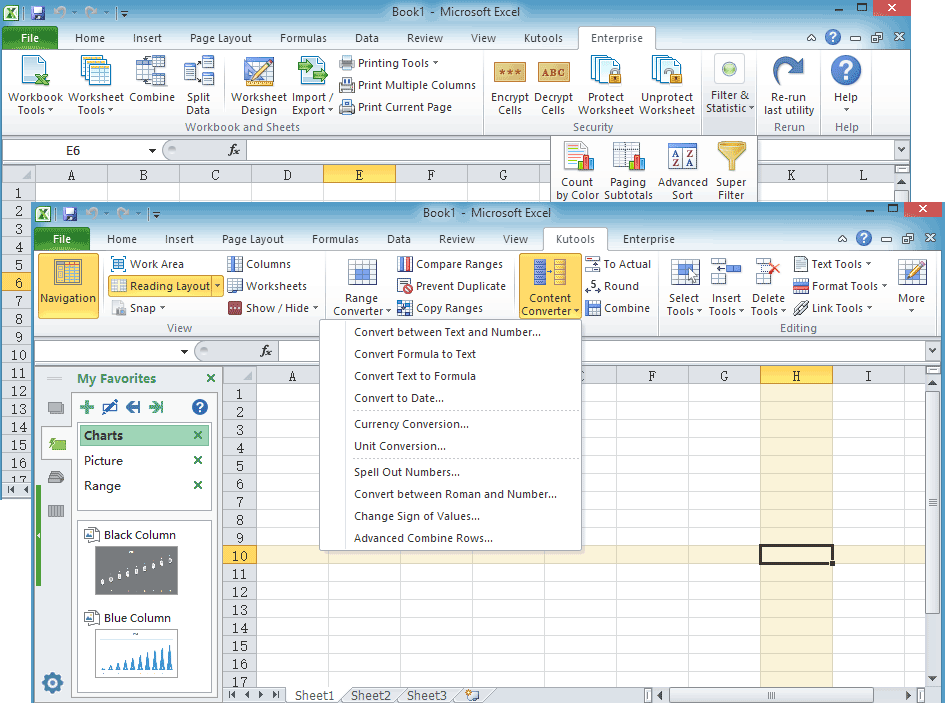## Quickly round cell values without formula in Excel

### Kutools for Excel

Boosts Excel With 300+
Powerful Features

Sometimes we may need to round the cell values up or down in Excel. But using the roundup or round down formula, they won’t change the actual values of cells after rounding. With Round utility of Kutools for Excel, you can easily apply the following operations:

• Rounding a range of values and display the actual values without formula
• Rounding up a range of values and display the actual values without formula
• Rounding down a range of values and display the actual values without formula
• Rounding a range of values to even and display the actual values without formula

#### Click Kutools >> Round. See screenshot: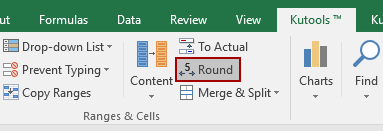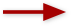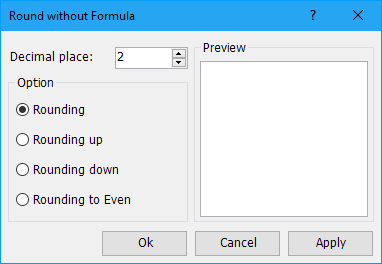#### Usage:

1. Select the range that you want to round the numbers.

2. Then apply the utility by clicking Kutools > Round, and specify the rounding option and decimal place digits in the Round Without Formula dialog box. See screenshot: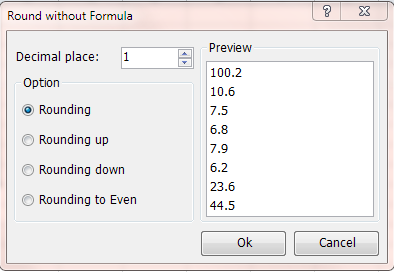3. Click Ok to apply the operation.

#### Notes:

Rounding: the same as the =ROUND(number, num_digits) formula. For example, if the cell value is 23.56, and you set the decimal place digits to 1, and the value will be 23.6 after rounding. See screenshots: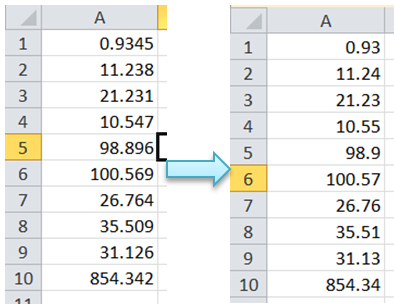Rounding Up: the same as the =ROUNDUP(number, num_digits) formula. For example, if the cell value is 45.23, and you set the decimal place digits to 1, and the value will be 45.3 after rounding up. See screenshots: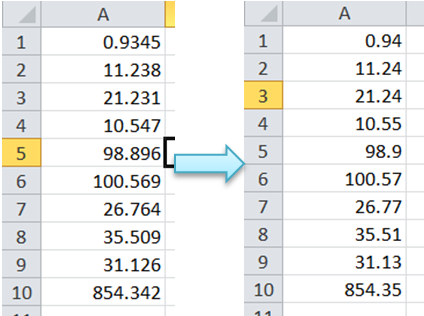Rounding Down: the same as the =ROUNDDOWN(number, num_digits) formula. For example, if the cell value is 38.49, and you set the decimal place digits to 1, and the value will be 38.4 after rounding down. See screenshots: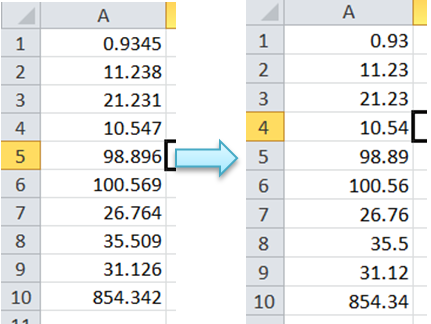Rounding to Even: For example, if the cell value is 7.55, and you set the decimal place digits to 1, the value will be 7.6 after rounding. But if the cell value is 8.65, the cell value will be 8.6 after rounding. See screenshots: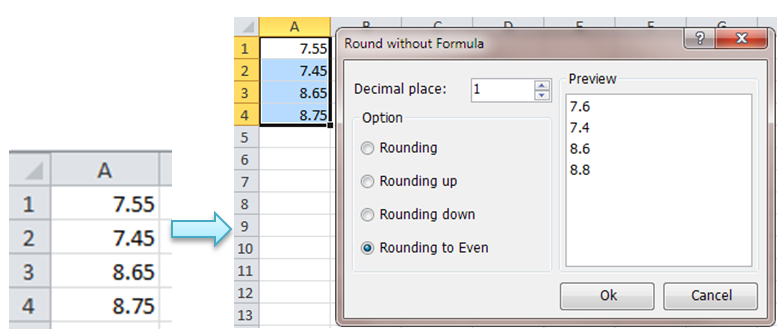#### Demo: Round / Round down / Round up a range of cells

Kutools for Excel includes more than 300 handy tools for Excel, free to try without limitation in 30 days. Download and Free Trial Now!

Productivity Tools Recommended
The following tools can greatly save your time and money, which one is right for you?
Office Tab: Using handy tabs in your Office, as the way of Chrome, Firefox and New Internet Explorer.
Kutools for Excel: More than 300 Advanced Functions for Excel 2021, 2019, 2016, 2013, 2010, 2007 and Office 365.

### Kutools for Excel

The functionality described above is just one of 300 powerful functions of Kutools for Excel.

Designed for Excel(Office) 2021, 2019, 2016, 2013, 2010, 2007 and Office 365. Free download and use for 30 days.